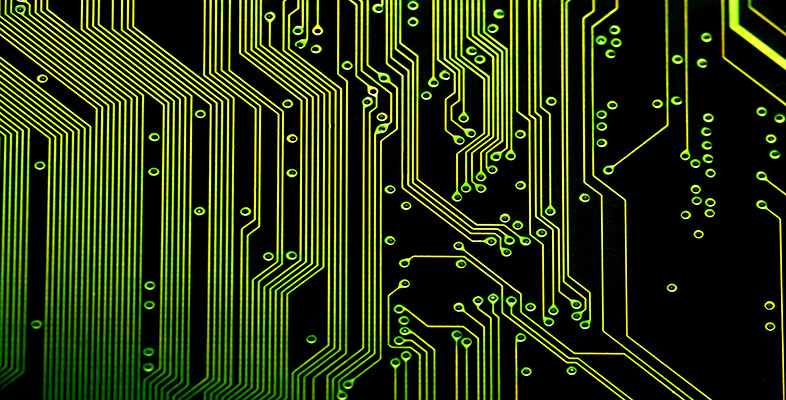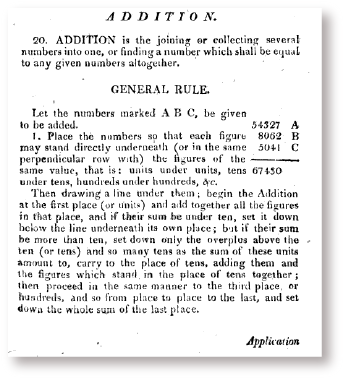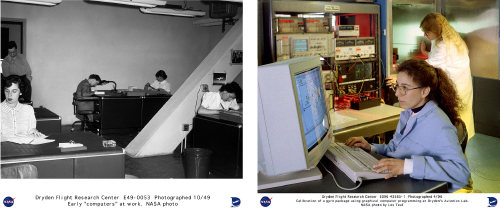Digital & Computing

### Become an OU studentIntroduction to computational thinking

Start this free course now. Just create an account and sign in. Enrol and complete the course for a free statement of participation or digital badge if available.

# 1 Computational thinking and automation

To state more precisely what computational thinking is, we need to consider the ideas of an algorithm and a computational problem. We will think about these ideas in the next activity.

## Activity 1 Algorithms and computational problems

Look at Figure 2. This shows page 18 of John Gough’s Practical Arithmetick in Four books, which is a tutorial maths book first published in 1767. The text under the heading ‘General Rule’ gives a description of a process for adding together three non-negative integers, each written as a finite sequence of digits. (The fact that three numbers are added is rather arbitrary: the process would work just as well for adding together two numbers or indeed any number of numbers.)Figure 2 Instructions for column addition (Gough, 1767)

Explain whether this textual description is an algorithm according to the following description of an algorithm.

An algorithm is a solution to a problem which has three properties:

• an algorithm consists of a step-by-step list of instructions
• an algorithm is a finite process (this means it is guaranteed to finish at some point)
• the algorithm should solve any instance of the problem that might arise.

### Discussion

First, we need to consider whether the text describes a step-by-step list of instructions. This is not always obvious. At some points it does and at some points it doesn’t.

At many points the text does provide instructions to be carried out sequentially, such as:

• ‘place the numbers so that each figure may stand directly underneath (…) the figures of the same value’
• ‘begin the addition at the first place’.

Although other parts of the instructions are not explicitly presented as a list of instructions, it is easy to see how the textual passage could be mapped onto such a list. In that list, certain instructions would be repeated for each of the columns involved (‘then proceed in the same manner … from place to place to the last’).

So, let’s assume that the text does have the first property. Does it also have the other two properties?

According to the second property, the process should be finite. This is indeed the case. The numbers being added will each have a finite number of digits, so only a finite number of columns will be involved, and the process only requires a small number of steps at each column.

Finally, the process does apply to any instance of the problem, where the ‘instances of the problem’ are all combinations of three integers, each represented by a finite sequence of digits in the range 0–9. The text leaves it implicit what instances the algorithm is intended to apply to.

The precise specification of the ‘instance of the problem’ can be thought of as part of the task of formulating a problem as a computational problem. By a computational problem, we mean "a problem that is stated sufficiently precisely such that one can attempt to write an algorithm to solve it."Figure 3 (a) Human computers working on test flight calculations at the predecessor of NASA in 1949 (b) two NASA employees in 1990 programming a computer so it can automatically calibrate aircraft equipment

Having met the ideas of algorithms and computational problems, let us state what computational thinking is not: computational thinking is not about us humans following an algorithm when carrying out the task of adding numbers on paper or in our head. It is not about thinking like a computer - rather, computational thinking is first and foremost thinking about computation. In particular, computational thinking consists of the skills to:

• formulate a problem as a computational problem
• construct a good computational solution (i.e. an algorithm) for the problem, or explain why there is no such solution.

A computational thinker can take a problem and state it sufficiently precisely for it to be potentially solvable by an algorithm. Once the problem has been stated in the right way, a computational thinker tries to construct an algorithm that solves it. A computational thinker won’t, however, be satisfied with just any solution: the solution has to be a ‘good’ one. We will discuss two specific properties of a good solution later on in this course: efficiency and correctness. Finally, computational thinking goes beyond finding solutions: if no good solution exists, one should be able to explain why this is so. This topic, however, takes us beyond the scope of this course into computability and computational complexity theory.

Most of the activities in this course involve watching extracts from Wing’s presentation ‘Computational Thinking and Thinking About Computing’. Remember to read through the activity before watching the video.